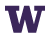Please submit the .Rmd file and all output files (.html, .pdf, etc.)

## Some quick problems

1. Create an object called myObject and assign it a value between 1 and 100

2. Add 13 to myObject, making sure the object itself stores the updated value

3. Is myObject divisible by 2? by 3? by 13? by 21? Use R code to get the answer.

4. How many times can 5 fit in myObject?

5. Add myObject to every element of a vector with values 1, 2, 3, 4, and 5

6. Fix this code (without changing any numbers) to get it to return 8

5+3^3%/%2

## R Markdown Practice

Create an R markdown document with the following components:

• A custom title (i.e., not “Untitled”) and your name

• Change the theme from the default theme

• An in-line R calculation

• Write the equation for the probability density function the normal distribution (R Markdown renders LaTeX style math, so Google will be your friend here!) $\frac{1}{\sigma\sqrt{2\pi}}e^{-\frac{1}{2}\left(\frac{x-\mu}{\sigma}\right)^2}$

• A block of R code (whatever you want) that is displayed, run, but does not show output in the rendered document

• A block of R code that is displayed, but is not run and thus does not show output in the rendered document

• A block of R code that is not shown, but produces output in the rendered document

• An ordered list

1. This
2. Is
3. an ordered
4. list!
• An unordered list

• this is an

• unordered list!
• A link to an external website like UW’s website

• A plot, 5in $$\times$$ 7in that is right-aligned in the rendered document (hint: if you need help generating a random plot, copy example code at the bottom of ?plot)

• A pictureUW Logo

• Bold text
• Italicized text
• Look online for an R package that might be useful for you in the future! This can be a package for conducting a specific type of analysis, creating certain figures, or anything else! Load the package in your markdown script and give me a two-sentence overview of the package.
• Bonus:
• Underlined text
• Red text
• White text with a red background
• Render a MS Word document
• Render a PDF document